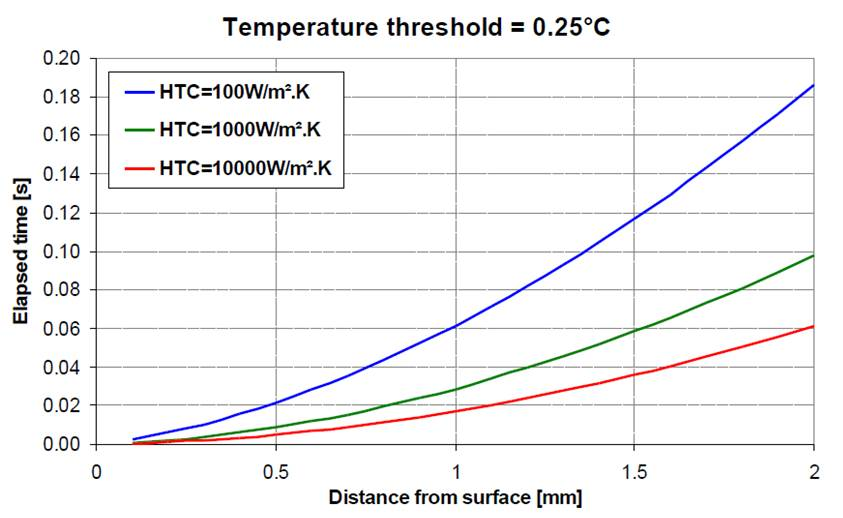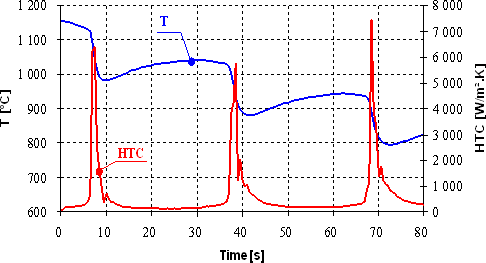Majority of studies in the Heat Transfer and Fluid Flow Laboratory is based on experimental measurements. Temperature histories in test samples are recorded during laboratory experiments. Similar data are obtained during plant measurements. The measured temperatures are input for evaluation where surface temperatures, heat fluxes and heat transfer coefficients must be computed. Inverse task means finding of the heat transfer coefficient (or heat flux) on the body surface. One or more temperature records at points (sensor positions) inside the body are known from the experiment.

## Introduction

Cooling intensity is essential parameter when temperature field in a cooled body shall be determined. The heat transfer tests showed that there is no simple explicit link between coolant flow rate and cooling intensity. Many other parameters such as flow velocity, surface roughness, or droplet size in case of spray cooling play important role. Simple estimation of the cooling intensity from flow rate can be affected by significant errors.

Inverse heat conduction problem IHCP is used to obtain cooling intensity given by physical quantity of heat transfer coefficient HTC when surface temperatures during cooling are not available. In such cases temperature history at one or more interior locations are measured to obtain input data for IHCP.

## Heat Equation

The heat transfer coefficient HTC is boundary condition for heat equation solving temperature field inside a cooled solid body.

Apart from two boundary conditions for each coordinate chosen from:

one initial condition

has to be known to solve the temperature field inside the body. There is no general analytical solution of the equation and must be solved numerically.

## Inverse Heat Conduction Problem IHCP

The calculation procedure of IHCP is reverse to calculation procedure of heat equation and is realized numerically. While temperature field inside the cooled body is calculated from boundary conditions by solving heat equation, the IHCP uses internal temperatures as input to get boundary condition (surface heat flux and HTC). The temperature during cooling is gained by measurement by thermocouples inside a body. The IHCP is mathematically ill-posed problem sensitive to errors in input data. To stabilize the IHCP the Beck’s sequential algorithm based on sequential estimation of time varying boundary condition and use of future time steps data is used.

To determine boundary condition HTC in given time, the measured temperatures are compared with the computed temperatures by using future time steps and number of thermocouples. The SSE denotes the sum of square errors that has to be minimized.

The surface heat flux that corresponds with minimization of SSE is:

The sensitivity coefficient represents the temperature rise at the thermocouple location per unit surface heat flux. When surface heat flux in given time is determined, the corresponding surface temperature is calculated from surface heat flux by using heat equation. The HTC is computed as

The coolant temperature is measured continuously during the experiment thus it is known for each time step. Once the heat transfer coefficient at given time is computed, the time index is incremented by one, and the procedure is repeated for the next time step.

## Temperature Measurement

Experimental work is essential for IHCP solution. The temperature history needed as IHCP input is measured by thermocouples inbuilt in an investigated body while the body is experimentally cooled under required conditions.The sensor with inbuilt thermocouple was developed in Heat Transfer and Fluid Flow Laboratory to enhance temperature measurement technique

It was shown that measurement technique including thermocouple embedding is critical for accuracy of IHCP results. The thermocouple type and way of its embedding together with measurement noise play significant role. The thermocouple has to be taken into account in numerical model of a body because its temperature profile is disturbed by inserted thermocouple.

Even if the thermocouple is involved into the calculation of temperature field, the boundary conditions at the surface do not need to be able to determine e.g. in cases when the measured temperature gradients are comparable to measurement noise or when the measurement frequency is too small in view of changes in boundary conditions.The graph answered the question at which distance from surface and in what time delay is temperature difference of 0.25°C for three surface cooling intensities given by HTC

For measurements with fast changes in boundary conditions a new combined inverse method was developed. This method is a mix of Downhill Simplex Optimization Method and Sequential Function Specification Method. This method gives more accurate results and suppresses a noise in computed results.Example of computed heat transfer coefficient HTC from measured temperature history T inside a steel plate using Sequential Function Specification Method

## Data Evaluation

The temperatures measured during cooling experiment are affected by noise and errors caused by transmission of signal from the thermocouple to the temperature. The data filtration becomes important for ill-posed problems such as IHCP. Therefore the measured temperatures are filtered before they are used as IHCP input. There are various filter techniques. Some of them use filtration in the time domain; some cut high frequencies in a frequency domain; and others approximate the measured data using a function.

The heat transfer coefficient, surface temperature and surface heat flux in dependency on cooling time and position is calculated from temperatures measured by thermocouples after filtration. This data is used to get temperature field in a body in any cooling time and is basis for cooling units design.The graph shows temperature at a body surface Ts and heat transfer coefficient HTC during a spray cooling experiment calculated by using IHCP; temperature Tm measured by thermocouple placed under the body surface is IHCP input